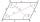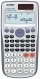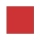# Parallelogram

Calculate the area and perimeter of a parallelogram whose two sides are long a=24 cm b=22 cm and height ha = 6 cm long.

Result

p =  92 cm
S =  144 cm2

#### Solution:Leave us a comment of example and its solution (i.e. if it is still somewhat unclear...):Be the first to comment!## Next similar examples:

1. Parallelogram - sideCalculate the length of the side of a parallelogram whose area is 175 dm2 and height to that side is 24.2 dm long.
2. ParallelogramCalculate the missing side of a parallelogram, if you know the perimeter o and one side: a) o = 7.2 cm; b = 1.8 cm b) o = 5.4 cm; a = 1.9 cm
3. ParallelogramIn the parallelogram we know one internal angle 67°33`. Calculate the other internal angles.
4. Circle - easy 2The circle has a radius 6 cm. Calculate:
5. AnnulusThe radius of the larger circle is 8cm, the radius of smaller is 5cm. Calculate the contents of the annulus.
6. Right triangleRight triangle legs has lengths 630 mm and 411 dm. Calculate the area of this triangle.
7. 22/7 circleCalculate approximately area of a circle with radius 20 cm. When calculating π use 22/7.
8. Perimeter of squareThe square has a circumference 17cm. What is its area?
9. MidpointsTriangle whose sides are midpoints of sides of triangle ABC has a perimeter 45. How long is perimeter of triangle ABC?
10. Clock handsThe second hand has a length of 1.5 cm. How long does the endpoint of this hand travel in one day?
11. Circle - simpleThe circumference of a circle is 930 mm. How long in mm is its diameter?
12. Bicycle wheelAfter driving 157 m bicycle wheel rotates 100 times. What is the radius of the wheel in cm?
13. Without 2Without multipying, tell whether the product 0.644 x 0.25 will be greater than 1 or less than 1? Explane how you know. Then find the product.
14. SickSick Marcel already taken six tablets, which was a quarter of the total number of pills in the pack. How many pills were in the pack?
15. Equation 11Solve equation: ?
16. SimplifySimplify powers multiplication: (3+22)(5-42)
17. Double 5Peter was thinking of a number. Peter doubles it and gets an answer of 8.6. What was the original number?